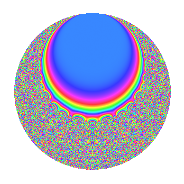# Properties

 Label 12.20.bLevel 12 Weight 20 Character orbit b Rep. character $$\chi_{12}(11,\cdot)$$ Character field $$\Q$$ Dimension 36 Newform subspaces 1 Sturm bound 40 Trace bound 0

# Related objects

## Defining parameters

 Level: $$N$$ = $$12 = 2^{2} \cdot 3$$ Weight: $$k$$ = $$20$$ Character orbit: $$[\chi]$$ = 12.b (of order $$2$$ and degree $$1$$) Character conductor: $$\operatorname{cond}(\chi)$$ = $$12$$ Character field: $$\Q$$ Newform subspaces: $$1$$ Sturm bound: $$40$$ Trace bound: $$0$$

## Dimensions

The following table gives the dimensions of various subspaces of $$M_{20}(12, [\chi])$$.

Total New Old
Modular forms 40 40 0
Cusp forms 36 36 0
Eisenstein series 4 4 0

## Trace form

 $$36q - 47880q^{4} + 21771144q^{6} - 809473644q^{9} + O(q^{10})$$ $$36q - 47880q^{4} + 21771144q^{6} - 809473644q^{9} - 4162950000q^{10} - 2268767880q^{12} - 25913431656q^{13} + 791689296672q^{16} + 2852271258192q^{18} - 1650825908424q^{21} + 7247870602416q^{22} + 23719435803936q^{24} - 118879690462380q^{25} - 7629081673968q^{28} + 37456727138640q^{30} + 529254701828640q^{33} - 248241675948480q^{34} - 1177548745309416q^{36} + 1847838600168120q^{37} - 40433256181440q^{40} - 2709671988768336q^{42} - 4365410114088000q^{45} + 17206125364795104q^{46} + 1561106052682272q^{48} - 49399737041580084q^{49} + 16558671470242896q^{52} + 40087412948587896q^{54} + 44803667812766472q^{57} - 34645536255764880q^{58} + 50260381053157440q^{60} + 174742350239997144q^{61} - 139766490424918656q^{64} - 87772097712685968q^{66} - 957190369821780672q^{69} + 244663962371376480q^{70} + 54134736422921280q^{72} - 1445122541399481432q^{73} + 2744130151937256048q^{76} + 1125465917961999024q^{78} + 1033298241353042436q^{81} + 1064985418859680800q^{82} + 1920709484261873424q^{84} - 1802899595088057600q^{85} - 9209792381828477760q^{88} - 5194944179528261040q^{90} + 6719944190337314136q^{93} - 3835186345170303552q^{94} - 11947184361208227456q^{96} + 12765226159947004488q^{97} + O(q^{100})$$

## Decomposition of $$S_{20}^{\mathrm{new}}(12, [\chi])$$ into newform subspaces

Label Dim. $$A$$ Field CM Traces $q$-expansion
$$a_2$$ $$a_3$$ $$a_5$$ $$a_7$$
12.20.b.a $$36$$ $$27.458$$ None $$0$$ $$0$$ $$0$$ $$0$$